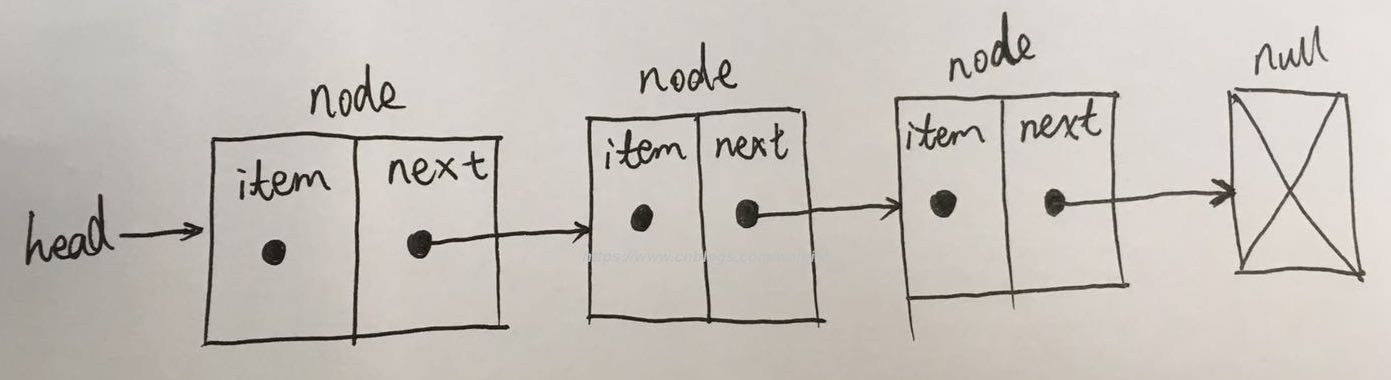js数据结构与算法--单链表的实现与应用思考链表的实现

/**

* 链表节点，链表中的项，链表中的节点

*/

export class Node {

constructor(element, next = null) {

this.element = element // 链表中节点的值

this.next = next // 指向列表中下一个节点项的指针

}

}

import { Node } from './linkednode'

/**

* 链表类

*/

constructor() {

this.length = 0 // 存储链表中列表项的数量

}

/**

* 向列表尾部添加一个新的项

* @param {*} element 需要添加至链表中的节点项

*/

append(element) {

let node = new Node(element) // 将新项创建为符合链表结构的列表项

// 链表中的元素为空

} else {

let current = this.head // 将第一个节点的引用赋值给当前项

// 循环列表，直到找到最后一项

while (current.next) {

current = current.next

}

// 找到最后一项，将其next赋为node，建立链接

current.next = node

}

this.length++ // 更新链表的长度

}

/**

* 向列表的特定位置插入一个新的项

* @param {*} position 插入链表中的位置

* @param {*} element 需要添加的节点

*/

insert(position, element) {

let node = new Node(element)

// 检查越界

if (position > -1 && position <= this.length) {

let previous

let index = 0

// 在第一个位子添加

if (position === 0) {

node.next = current

} else {

// 需要找到特定的位子，将想要插入的元素放在previous节点和current节点之间

while (index++ < position) {

// previous 将是对想要插入新元素的位置之前一个元素的引用

previous = current

// current 对想要插入新元素的位置之后一个元素的引用

current = current.next

}

node.next = current

previous.next = node

}

this.length++

return true

} else {

return false

}

}

/**

* 移除指定位置的节点元素,并返回移除的项

* 如果超出了链表的范围，则返回null

* @param {Int32Array} position 链表中的位置

*/

removeAt(position) {

// 检查越界

if (position > -1 && position < this.length) {

let previous // 先前一个节点

let index = 0 // 当前节点的位子

// 移除第一项

if (position === 0) {

} else {

while (index++ < position) {

previous = current

current = current.next

}

// 将previous 与 current 的下一项链接起来：跳过current，从而移除它

// 当前元素会丢弃在计算机内存中，等待GC清除

previous.next = current.next

}

this.length-- // 链表元素数量减1

return current.element // 返回移除的项

} else {

return null // 如果超出了链表的范围，则返回null

}

}

/**

* 从列表中移除一项

* 先找出元素的索引项，再根据索引移除元素

* @param {*} element 列表中的元素

*/

remove(element) {

let index = this.indexOf(element)

return this.removeAt(index)

}

/**

* 返回元素在列表中的索引。如果列表中没有该元素则返回-1

* @param {*} element 元素

*/

indexOf(element) {

let index = 0 // 计算位置数

while (current) {

if (element === current.element) {

return index

}

index++

current = current.next

}

return -1

}

/**

* 判断是否为空链表

* 空链表返回true，非空(链表长度大于0)返回false

*/

isEmpty() {

return this.size() === 0

}

/**

* 返回链表包含的元素个数。与数组的length属性类似

*/

size() {

return this.length

}

/**

* 获取链表的表头节点

* 但是，我们需要实现在类的外部循环访问列表。

* 此时，就需要提供获取类的第一个元素的方法

*/

}

/**

* 由于列表项使用了Node类，需要重写toString方法，让其只输出元素的值。

*/

toString() {

let string = ''

while (current) {

string += current.element + (current.next ? '-->' : '')

current = current.next

}

return string

}

}

链表的应用思考

1. 链表中的元素是不连续的，而数组中的元素是连续的。

2. 链表添加或移除元素的时候不需要移动其他元素，而数组需要。

3. 链表需要指针，而数组不需要。

4. 链表需要从表头开始迭代列表直到找到所需要的元素。数组则可以直接访问任何位置的任何元素。

5. 两者都具有动态性。关于数组动态性。数组在js中是一个可以修改的对象，添加移除元素，它会动态的变化，但是成本很高，需要移动元素。在大多数语言中（比如C和Java），数组的大小是固定的，想添加元素就要创建一个全新的数组，不能简单地往其中添加所需的元素。

github: weiqinl

posted @ 2018-07-02 15:22  weiqinl  阅读(692)  评论(0编辑  收藏  举报